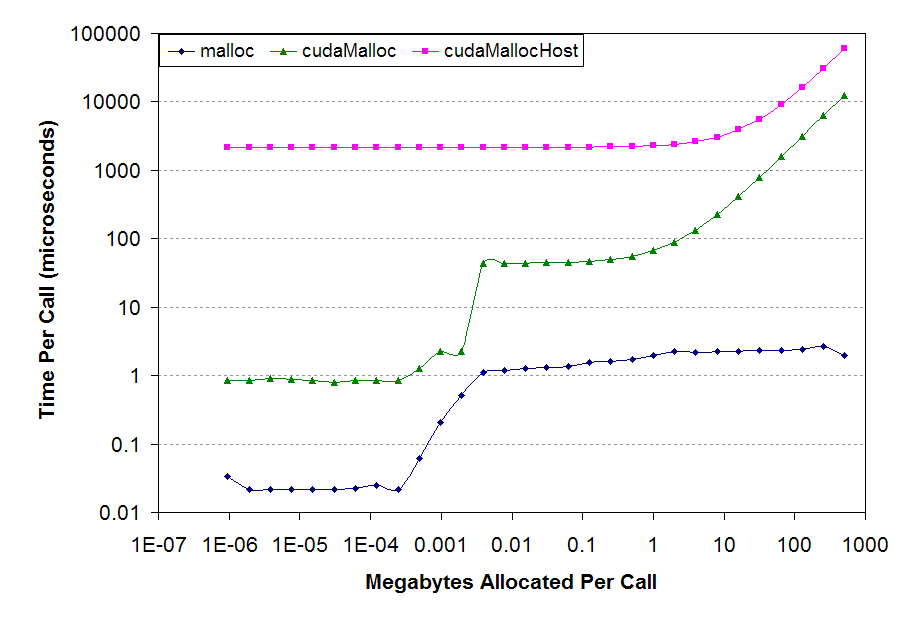# Cuda编程102

Cuda程序性能提升

Cuda为程序员使用GPU进行异构计算提供了抽象良好的编程模型。但正如同编写CPU程序时需注意局部性、缓存等硬件特性以获得更好地性能，为了更好地挖掘GPU的性能，我们在GPU程序中也需注意GPU特有的访存、执行、数据传输等方面的特性并进行相应的优化。

# GPU的内存层级

• Register Files
• L1 / Shared Memory*
• L2 Cache
• Global Memory*

__global__ void
multiply_without_shared(float* A, float* x, float* y, int N, int M) {
int idx = blockIdx.x * blockDim.x + threadIdx.x;
if (idx >= N) return;
int sum = 0;
for(int i = 0; i < M; ++i) {
sum += x[i] * A[i * M + idx];
}
y[idx] = sum;
}

__global__ void
multiply_with_shared(float* A, float* x, float* y, int N, int M) {
extern __shared__ float shared_x[];
for(int i = 0; i < M; ++i) {
shared_x[i] = x[i];
}
}
int idx = blockIdx.x * blockDim.x + threadIdx.x;
if (idx >= N) return;
int sum = 0;
for(int i = 0; i < M; ++i) {
sum += shared_x[i] * A[i * M + idx];
}
y[idx] = sum;
}


# GPU的分支语句对性能的影响

if (threadIdx.x % 2 == 0) {
// Some work
} else {
// Other work
}


switch (threadIdx.x) {
case 0: ...
case 1: ...
...
case 126: ...
case 127: ...
}


# GPU访存pattern对性能的影响

__global__ void
add_coalesced(float* A, float* B, float* C, int N) {
int idx = blockIdx.x * blockDim.x + threadIdx.x;
if (idx >= N) return;
C[idx] = A[idx] + B[idx];
}

__global__ void
add_striped(float* A, float* B, float* C, int N) {
int idx = blockIdx.x * blockDim.x + (threadIdx.x % 32) * 32 + (threadIdx.x / 32);
if (idx >= N) return;
C[idx] = A[idx] + B[idx];
}


# CPU、GPU间数据传输，PCIe，page-lock内存

CPU、GPU间的数据传输通过PCIe进行传输，目前服务器上常见的16x接口的理论带宽上限是16GB/s。CPU、GPU之间的所有数据传输均通过DMA完成，这就要求CPU侧的内存必须是page-lock的，而通常情况下我们向系统申请得到的内存均是pageable的，这就导致了当我们调用cudaMemcpy从CPU向GPU传输数据时，cudaMemcpy将执行以下判断：

• 若CPU内存是cuda runtime认为page-lock的，则直接发起DMA传输
• 若CPU内存是普通的pageable内存，则分配一段page-lock内存，将数据进行一次内存到内存的拷贝，再发起DMA传输• 尽可能少传，宁可用计算换传输量
• 如果使用了cudaMallocHost，尽可能重复使用以降低平均分配成本